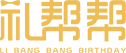• A
• B
• C
• D
• E
• F
• G
• H
• J
• K
• L
• M
• N
• P
• Q
• R
• S
• T
• W
• X
• Y
• Z

# 钟汉良1130过生日，粉丝送上最暖生日礼物？11月30日是钟汉良的生日，每年的这一天，全国各地的粉丝都会聚集起来为他庆生，自然少不了各种形式的生日祝福，做蛋糕，做手工，剪MV，画画……在这之中，有一种礼物格外的暖心。“读书不一定改变命运，但一定能丰富人生。”助学鞋盒礼物鞋盒礼物

“公益是很平常的事情，是大家的事情……作为艺人，我觉得做公益慈善活动有一个最大的好处，就是利用我的身份影响那些支持者，让他们都能来关心关注公益慈善事业。” ——钟汉良\$(function(){ if( \$('.flower-l-content-text').html().indexOf('[AD]')>0 ){ \$('.flower-l-content-text').html(function(i, h){ if( h.indexOf('[AD]') ) h = h.replace( '[AD]', \$('#sp1').html() ); if( h.indexOf('[AD]') ) h = h.replace( '[AD]', \$('#sp2').html() ); if( h.indexOf('[AD]') ) h = h.replace( '[AD]', \$('#sp3').html() ); return h; }); } else{ var p = \$('.flower-l-content-text p').length; \$('.flower-l-content-text p').eq( Math.ceil(p*0.2) ).after( \$('#sp1').html() ); \$('.flower-l-content-text p').eq( Math.ceil(p*0.5) ).after( \$('#sp2').html() ); \$('.flower-l-content-text p').eq( Math.ceil(p*0.8) ).after( \$('#sp3').html() ); } });Roselove千娇百媚花束Roselove一笑倾城花束Roselove娉婷袅袅花束## 给男朋友惊喜要拿准送惊喜的时机